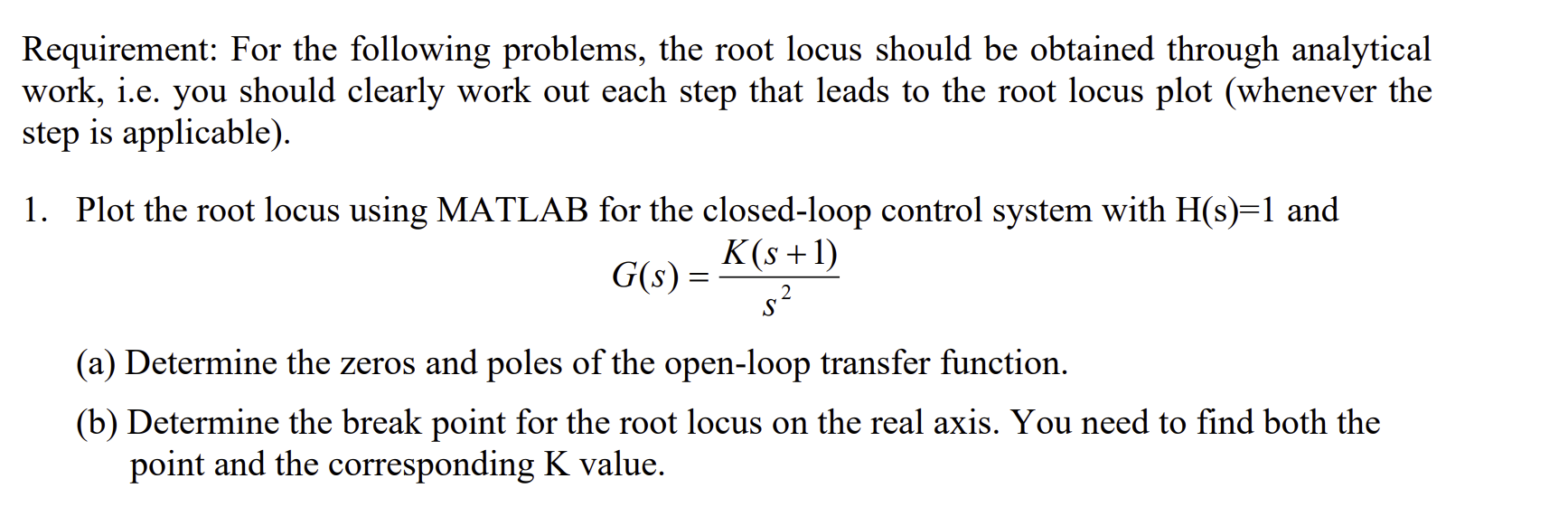Home / Expert Answers / Electrical Engineering / requirement-for-the-following-problems-the-root-locus-should-be-obtained-through-analytical-work-pa904

# (Solved): Requirement: For the following problems, the root locus should be obtained through analytical work, ...Requirement: For the following problems, the root locus should be obtained through analytical work, i.e. you should clearly work out each step that leads to the root locus plot (whenever the step is applicable). 1. Plot the root locus using MATLAB for the closed-loop control system with and (a) Determine the zeros and poles of the open-loop transfer function. (b) Determine the break point for the root locus on the real axis. You need to find both the point and the corresponding value.

We have an Answer from Expert